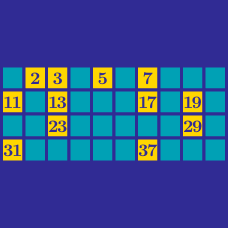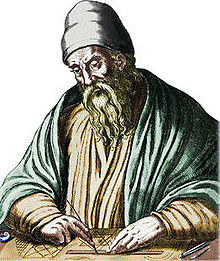Number Theory

# Prime Numbers: Level 4 ChallengesA common flawed presentation of Euclid's proof of the infinitude of primes is as follows:

Assume there are only a finite number of primes $p_1,p_2,\ldots,p_n$. Let $N$ be the product of all of those primes, add to it $1$ and you get a new prime number since it isn't divisible by any of the primes we listed at first. Contradiction! $\Rightarrow\Leftarrow$ Therefore, there is an infinite number of primes.

Let $q_1, q_2, q_3, \ldots$ be the list of all primes (in ascending order). Your mission is to find the smallest value of $q_1 q_2 q_3 \ldots q_n + 1$ that is not a prime.

Let $A={102^1, 102^2, 102^3, \cdots}$. How many primes $p$ are there such that $A$ has at least one element $a$ such that $a \equiv -1 \text{ (mod p)}$?

For example, one such prime is $103$, because $102^1 \equiv -1 \text{ (mod 103)}$.

$\Large \frac{d}{dx} n \neq 0?$

In calculus, when you take the derivative of a constant you get zero as an answer. In number theory, there is something called the arithmetic derivative which allows you to differentiate a number and get a nonzero answer. The arithmetic derivative works as follows.

Where $n'$ denotes the arithmetic derivative of $n$:

$p' = 1$ for all primes $p$

$(ab)'=a'b+ab'$

$0'=1'=0$

For example, $6'=(2\times3)'=(2')(3)+(2)(3')=(1)(3)+(2)(1)=5$

The double arithmetic derivative, denoted by $n''$, is simply defined by $n''=(n')'$.

Find the sum of all positive integers $n<100$ such that $n''=1$

$\Huge { \sum_{m=1}^{2^{820}} } \large \left \lfloor \left \lfloor \frac{820}{1 + \displaystyle \sum_{j=2}^m \left \lfloor\frac{(j-1)!+1}{j} -\left \lfloor\frac{(j-1)!}{j}\right \rfloor \right \rfloor }\right \rfloor ^{1/820} \right \rfloor = \ ?$

You may use this List of Primes as a reference.

Note: By definition, $\displaystyle \sum_{a=b+1}^b 1 = 0$.

###### This summation is rather infamous.

What is the smallest prime number that cannot be written in the form $\dfrac{pq + 1}{p + q}$, where $p$ and $q$ are prime numbers?

For example, $2$ can be written as $\dfrac{3*5 + 1}{3 + 5}$.

×